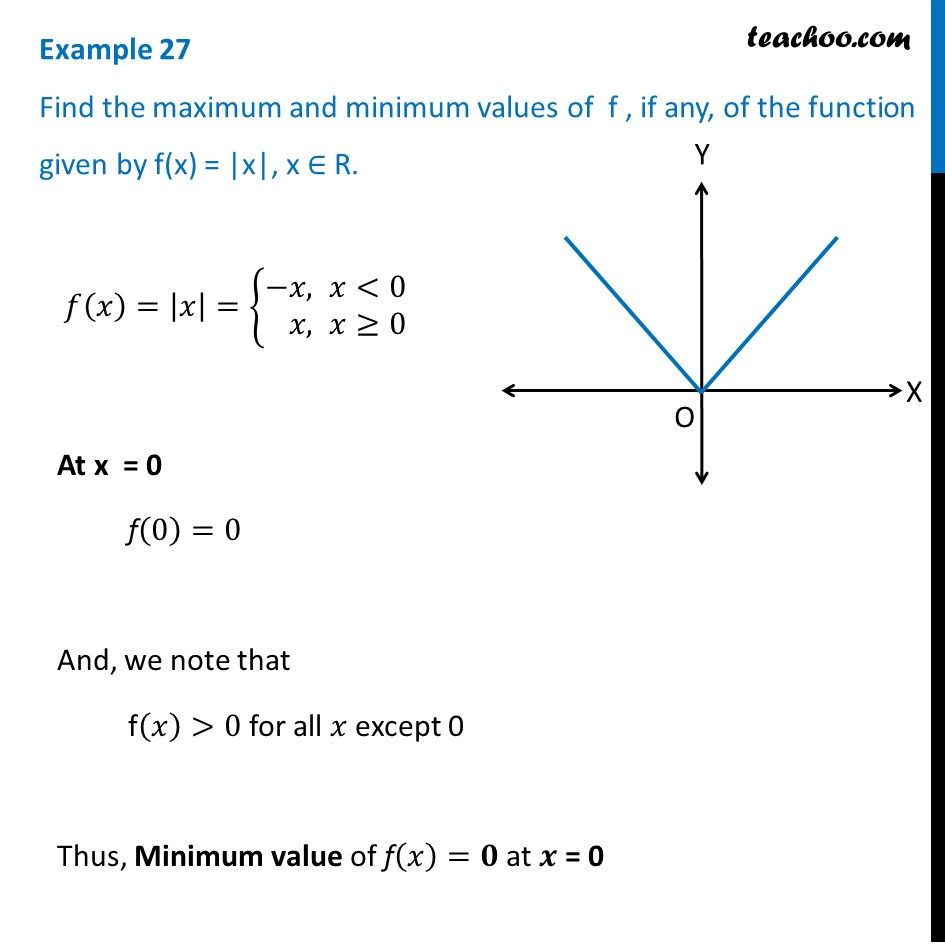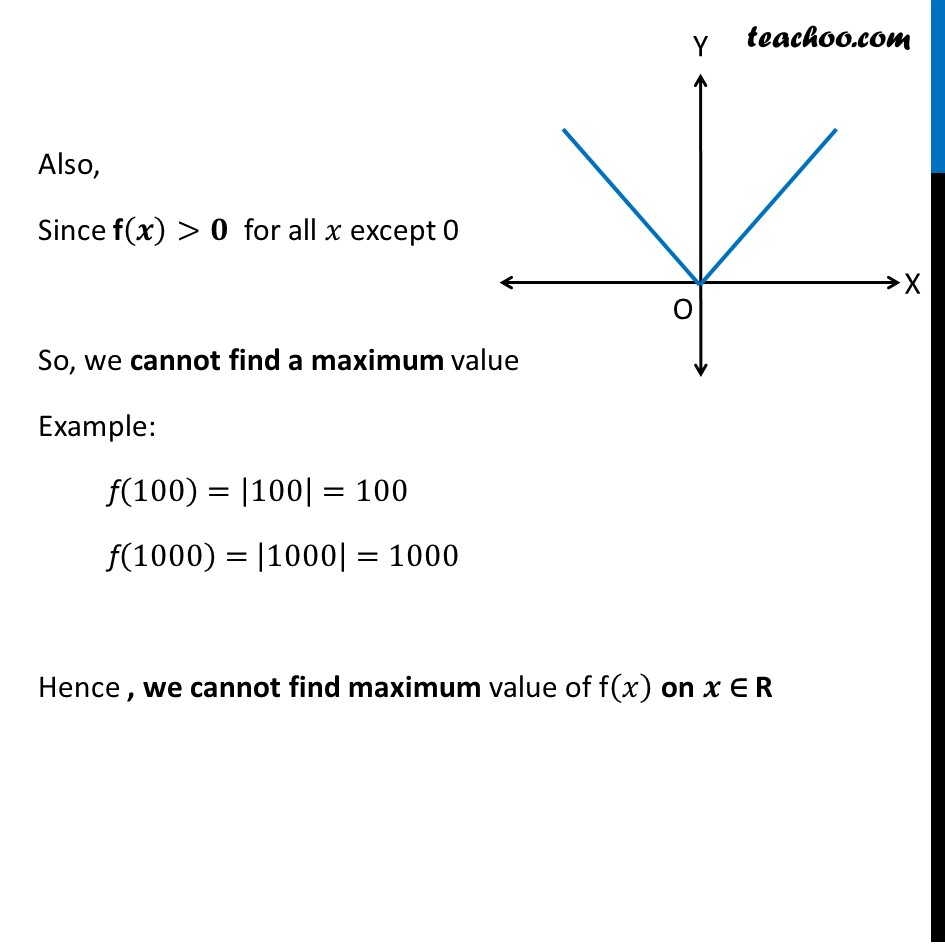1. Chapter 6 Class 12 Application of Derivatives (Term 1)
2. Serial order wise
3. Examples

Transcript

Example 27 Find the maximum and minimum values of f , if any, of the function given by f(x) = |x|, x ∈ R. 𝑓(𝑥)=|𝑥|={█(−&𝑥, 𝑥<0@&𝑥, 𝑥≥0)┤ At x = 0 f(0)=0 And, we note that f(𝑥)>0 for all 𝑥 except 0 Thus, Minimum value of f(𝑥)=𝟎 at 𝒙 = 0 Also, Since f(𝒙)>𝟎 for all 𝑥 except 0 So, we cannot find a maximum value Example: f(100)=|100|=100 f(1000)= |1000|=1000 Hence , we cannot find maximum value of f(𝑥) on 𝒙 ∈ R

Examples

Chapter 6 Class 12 Application of Derivatives (Term 1)
Serial order wise